## Inserting and Missing Character

#### Inserting and Missing Character

Direction: Find the missing character in each of the following questions :

1. NA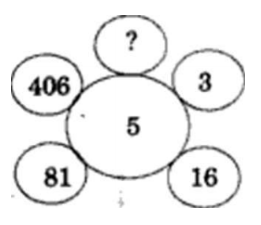1. 1
2. 731
3. 1625
4. 2031

1. Clearly, we have : (3 x 5) + 1 = 16 , (16 x 5) + 1 = 81 , (81 x 5) + 1 = 406
So, aliasing number = (406 x 5) + 1 = ?

##### Correct Option: D

Clearly, we have : (3 x 5) + 1 = 16 , (16 x 5) + 1 = 81 , (81 x 5) + 1 = 406
So, aliasing number = (406 x 5) + 1 = ?
? = 2031

1. NA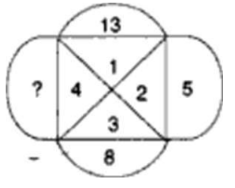1. 10
2. 11
3. 12
4. 13

1. The arrangement i* : 5 + 3 = 8 , 8 + 4 = 12 ,
So , ? + 1 = 13

##### Correct Option: C

The arrangement i* : 5 + 3 = 8 , 8 + 4 = 12 ,
So , ? + 1 = 13
? = 13 - 1 = 12
So. the missing number is 12.

1. NA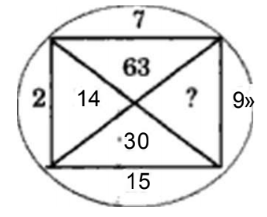1. 33
2. 145
3. 135
4. 18

1. Clearly, we have : 15 x 2 =30 , 2 x 7 = 14 , 7 x 9 = 63.
So, missing number = 9 x 1 5 = ?

##### Correct Option: C

Clearly, we have : 15 x 2 =30 . 2 x 7 = 14, 7 x 9 = 63.
So, missing number = 9 x 1 5 = ?
? = 135.

1. NA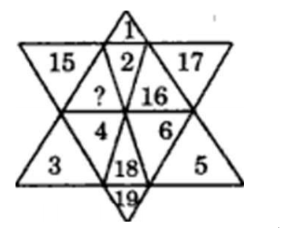1. 13
2. 14
3. 20
4. 21

1. The given figure contains numbers 1 to 6 in three alternate segments, the smaller number being towards the outside and the numbers 14 to 19 in the remaining three alternate segment s with the smulle- number towards the inside.

##### Correct Option: B

The given figure contains numbers 1 to 6 in three alternate segments, the smaller number being towards the outside and the numbers 14 to 19 in the remaining three alternate segment s with the smulle- number towards the inside.

1. NA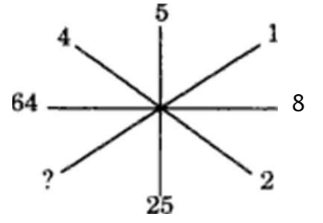1. 1
2. 2
3. 3
4. 4

1. The two ends of each line segment contain a number and its square.
We have , 22 = 4
52 = 25
82 = 64
So, missing number = 12 = ?

##### Correct Option: A

The two ends of each line segment contain a number and its square.
We have , 22 = 4
52 = 25
82 = 64
So, missing number = 12 = ?
? = 1